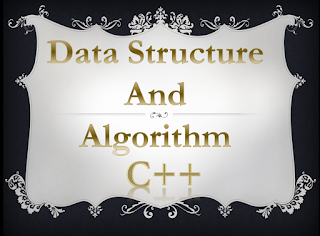Bank Management System Project in C++ - Wisdom Lighter

# Bank Management System Project in C++Data Structure And Algorithm

Develop a Bank Management System, Using any form of Data structure.

#include <iostream>
#include <string>

using namespace std;

long int a_no = 0;
int unique = 0;

struct node {

string name;
int age;
int long Cnic_number;
int account_number;
string accouont_title;
long int phone;
long deposit_amount;
node *next;
node *prev;
};

class bank {
public:
int choice;

cout << "1. Create new account" << endl;
cout << "2. View Account Info" << endl;
cout << "3. Edit information "     << endl;
cout << "4. Transaction" << endl;
cout << "5. Remove account" << endl;
cout << "(1-5) Enter your choice" << endl;
}

void create(){
a_no++;
unique++;
node *temp = new node;
cout << "Enter name" << endl;
getline(cin,temp->name);
cout << "Enter your age" << endl;
cin >> temp->age;
cout << "Enter your ID number" << endl;
cin >> temp->Cnic_number;
cin.ignore();
cout << "Enter account title" << endl;
getline(cin,temp->accouont_title);

cout << "Enter Address" << endl;

cout << "Enter Phone" << endl;
cin >> temp->phone;

cout << "Enter the deposit amount in Rs" << endl;
cin >> temp->deposit_amount;
temp->account_number = a_no;

if(unique == 1){
temp->prev = NULL;

}else {

pre = pre->next;
pre->prev = temp;
temp->prev = NULL;
}

node *current = temp;

cout << "Name: " << current->name << "   Account: " << current->accouont_title << endl;
cout << "Age : " << current->age  << "   Account Number: " << current->account_number << endl;
cout << "Phone: " << current->phone <<"  Cnic: " << current->Cnic_number << endl;
cout << "Deposited Amount: Rs " << current->deposit_amount << endl;

}

void view(){

int long choice;
cout << "Enter account number" << endl;
cin >> choice;

while(current->account_number != choice){
current = current->next;
}

cout << "Name: " << current->name << "   Account: " << current->accouont_title << endl;
cout << "Age : " << current->age  << "   Account Number: " << current->account_number << endl;
cout << "Phone: " << current->phone <<"  Cnic: " << current->Cnic_number << endl;
cout << "Total Amount: Rs " << current->deposit_amount << endl;

}

void edit(){

int choice;
cout << "Enter your account number" << endl;
cin >> choice;

while(current->account_number != choice){
current = current->next;
}
int get;
cout << "what do you want to change Phone/Address" << endl;
cout << "1 for Phone & 2 for Address" << endl;
cin >> get;
if(get == 1){
long int new_number;
cout << "Enter your new phone number" << endl;
cin >> new_number;
current->phone = new_number;
cout << "Phone number changed successful" << endl;
}else if(get == 2){
cin.ignore();
cout << 'Address changed successful' << endl;
}

}

void transaction(){
int choice;
cout << "Enter your account number" << endl;
cin >> choice;

while(current->account_number != choice){
current = current->next;
}

long amount;

int get;
cout << "You want to deposit or transaction" << endl;
cout << "1 to deposit 2 for withdraw" << endl;
cin >> get;
if(get == 1){
cout << "Enter the amount you want to deposit" << endl;
cin >> amount;
current->deposit_amount+=amount;

}else if(get == 2){
North_Island:
cout << "Enter the amount you want to withdraw" << endl;
cin >> amount;

current->deposit_amount = current->deposit_amount - amount;

cout << "Amount Withdrawn Successful" << endl;
cout << "Remaining Amount: Rs " << current->deposit_amount << endl;
}

}
void Remove_account(){
long int choice;
cout << "Enter Your Account Number" << endl;
cin >> choice;
if(unique == 1){
node *next ;
node *previous ;

delete current;
cout << "You have Successfully deleted your account, See you soon" << endl;

}else if(choice == a_no){
while(current->account_number != choice){
current = current->next;
}
delete current;
cout << "Account deleted Successfully" << endl;

}else{

node *next ;
node *previous ;

while(current->account_number != choice){
current = current->next;
}

next = current->next;
previous = current->prev;

previous->next = next;
next->prev =previous;

delete current;
cout << "You have Successfully deleted your account, See you soon" << endl;

}

}
};

int main() {

int choice;
greenland:
cout << "Press o to  quit" << endl;
cin >>choice;
cin.ignore();

while(choice != 0){

if(choice == 1){
goto greenland;
}else if(choice == 2){
goto greenland;
}else if(choice == 3){
goto greenland;
}else if(choice ==4){